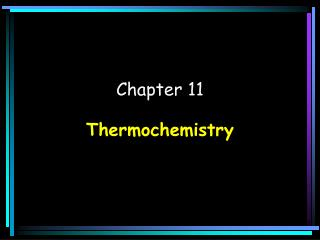DownloadDownload PresentationChapter 11

# Chapter 11

Télécharger la présentation## Chapter 11

- - - - - - - - - - - - - - - - - - - - - - - - - - - E N D - - - - - - - - - - - - - - - - - - - - - - - - - - -
##### Presentation Transcript

1. Chapter 11 Thermochemistry

2. H2O 1.01 x 2 2.02 16.00 x 1 +16.00 = 18.02 g Molar Heat of Fusion(Hfus): The energy needed to melt 1 mole of a substance. Water: ΔHfus= 6.01 kJ/mol ΔH values are positive because substances need added heat in order to melt. (Endothermic) 1 H Hydrogen 1.01 8 O Oxygen 16.00 Example: How much heat does it take to melt 35.0 g of ice? mol H2O 6.01 kJ 1 35.0 gH2O = 11.7 kJ x x mol H2O 1 g H2O 18.02 Calculator: 35.0 ÷ 18.02 x 6.01 = 11.67314095 kJ

3. Molar Heat of Solidification(Hsolid): The heat lost when 1 mole of a liquid freezes. Water: ΔHsolid= -6.01 kJ/mol ΔH values are negative because substances have to lose heat in order to freeze. (Exothermic)

4. H2O 1.01 x 2 2.02 16.00 x 1 +16.00 = 18.02 g Molar Heat of Vaporization(Hvap): The energy needed to vaporize 1 mole of a substance. Water: ΔHvap= 40.7 kJ/mol (Endothermic) 1 H Hydrogen 1.01 8 O Oxygen 16.00 Example: How much heat does it take to vaporize 35.0 g of water? mol H2O 40.7 kJ 1 35.0 gH2O = 79.1 kJ x x mol H2O 1 g H2O 18.02 Calculator: 35.0 ÷ 18.02 x 40.7 = 79.05105438 kJ

5. Molar Heat of Condensation(Hcond): The amount of heat released when 1 mole of a substance condenses. Water: ΔHcond = -40.7 kJ/mol (Exothermic)

6. Heat of Combustion(H): The heat released during a chemical reaction in which 1 mole of a substance is completely burned. Also called Molar Heat of Combustion (Exothermic)

7. Molar Heat of Solution(Hsoln): The heat change that results when 1 mole of a substance is dissolved in water. Molar heat of solution for calcium chloride ΔHsoln= -82.8 kJ/mol Example: Certain hot packs work by mixing calcium chloride with water. How much heat energy is produced if you mix 6.32 moles of CaCl2 in water? -82.8 kJ 6.32 molCaCl2 = -523 kJ x mol CaCl2 1 Calculator: 6.32 x -82.8 = -523.296 kJ

8. (Hess’s Law) Use Hess’s Law to calculate (∆H) for the reaction where graphite becomes diamond. C(graphite) C(diamond) ∆H =______ kJ Use the enthalpy changes for the combustion of graphite and diamond. 1) C(graphite)+ O2 (g) CO2(g) ∆H1 =-394 kJ CO2(g) 2) C(diamond)+ O2 (g) C(diamond)+ O2 (g) CO2(g) ∆H2 =- 396 kJ ∆H2 =+ 396 kJ Diamond is supposed to be a product so reverse 2nd reaction and change sign for ∆H. It is called Hess’s Law of Heat SUMMATION Graphite is supposed to be a reactant so leave 1st reaction alone. 1) C(graphite)+ O2 (g) CO2(g) ∆H1 =-394 kJ +  2) +2 C(graphite) C(diamond) ∆H =-394 + 396 =+ 2 kJ

9. Standard Heat of Formation: ∆Hf° = ∆Hf°(products) - ∆Hf°(reactants) 2 CH3OH (l) + 3 O2 (g) 2 CO2(g) + 4 H2O (l) -239 x 2 - 478 kJ 0.0 x 3 0 kJ -394 x 2 -788 kJ -286 x 4 -1,144 kJ + + - 478 kJ - 1,932 kJ (reactants) (products) ∆H°=∆Hf°(products) - ∆Hf°(reactants) ∆H° = - 1,932 kJ – (- 478 kJ) = - 1,454 kJ ∆H° = - 1,454 kJ